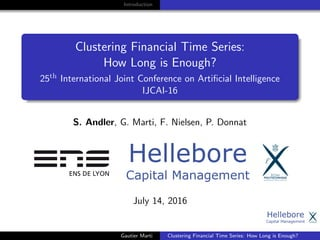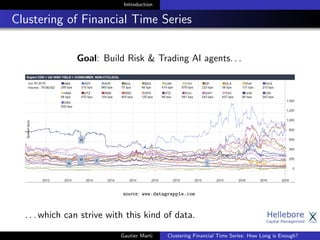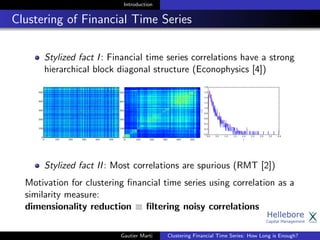Ce diaporama a bien été signalé.
Le téléchargement de votre SlideShare est en cours. ×

# Clustering Financial Time Series: How Long is Enough?

Publicité
Publicité
Publicité
Publicité
Publicité
Publicité
Publicité
Publicité
Publicité
Publicité
PublicitéChargement dans…3
×

## Consultez-les par la suite

1 sur 16 Publicité

# Clustering Financial Time Series: How Long is Enough?

IJCAI-16, New York, conference presentation of paper http://www.ijcai.org/Proceedings/16/Papers/367.pdf

Researchers have used from 30 days to several
years of daily returns as source data for clustering
financial time series based on their correlations.
This paper sets up a statistical framework to study
the validity of such practices. We first show that
clustering correlated random variables from their
observed values is statistically consistent. Then,
we also give a first empirical answer to the much
debated question: How long should the time series
be? If too short, the clusters found can be spurious;
if too long, dynamics can be smoothed out.

IJCAI-16, New York, conference presentation of paper http://www.ijcai.org/Proceedings/16/Papers/367.pdf

Researchers have used from 30 days to several
years of daily returns as source data for clustering
financial time series based on their correlations.
This paper sets up a statistical framework to study
the validity of such practices. We first show that
clustering correlated random variables from their
observed values is statistically consistent. Then,
we also give a first empirical answer to the much
debated question: How long should the time series
be? If too short, the clusters found can be spurious;
if too long, dynamics can be smoothed out.

Publicité
Publicité

### Clustering Financial Time Series: How Long is Enough?

1. 1. Introduction Clustering Financial Time Series: How Long is Enough? 25th International Joint Conference on Artiﬁcial Intelligence IJCAI-16 S. Andler, G. Marti, F. Nielsen, P. Donnat July 14, 2016 Gautier Marti Clustering Financial Time Series: How Long is Enough?
2. 2. Introduction Clustering of Financial Time Series Goal: Build Risk & Trading AI agents. . . source: www.datagrapple.com . . . which can strive with this kind of data. Gautier Marti Clustering Financial Time Series: How Long is Enough?
3. 3. Introduction Clustering of Financial Time Series Stylized fact I: Financial time series correlations have a strong hierarchical block diagonal structure (Econophysics ) Stylized fact II: Most correlations are spurious (RMT ) Motivation for clustering ﬁnancial time series using correlation as a similarity measure: dimensionality reduction ≡ ﬁltering noisy correlations Gautier Marti Clustering Financial Time Series: How Long is Enough?
4. 4. Introduction Challenge for the statistical practitioner The dilemma: the longer the time interval, the more precise the correlation estimates, but also the longer the time interval, the more unrealistic the stationarity hypothesis for these time series. Question: How does the clustering behave with statistical errors of the correlation estimates? How long is enough? 30 days? 120 days? 10 years? Gautier Marti Clustering Financial Time Series: How Long is Enough?
5. 5. Introduction A ﬁrst theoretical approach - simpliﬁed setting We consider the following framework: ﬁnancial time series ≡ random walks they follow a joint elliptical distribution (e.g. Gaussian, Student) parameterized by a correlation matrix the correlation matrix has a hierarchical block structure: Gautier Marti Clustering Financial Time Series: How Long is Enough?
6. 6. Introduction Simulations in the simpliﬁed setting Some inﬂuential parameters: clustering algorithm number of observations T number of variables N relative to T contrast between the correlations, and their values correlation estimator (e.g. Pearson, Spearman) 100 200 300 400 500 Sample size 0.0 0.2 0.4 0.6 0.8 1.0 Score Empirical rates of convergence for Single Linkage Gaussian - Pearson Gaussian - Spearman Student - Pearson Student - Spearman 100 200 300 400 500 Sample size 0.0 0.2 0.4 0.6 0.8 1.0 Score Empirical rates of convergence for Average Linkage Gaussian - Pearson Gaussian - Spearman Student - Pearson Student - Spearman 100 200 300 400 500 Sample size 0.0 0.2 0.4 0.6 0.8 1.0 Score Empirical rates of convergence for Ward Gaussian - Pearson Gaussian - Spearman Student - Pearson Student - Spearman Ratio of the number of correct clustering obtained over the number of trials as a function of T Gautier Marti Clustering Financial Time Series: How Long is Enough?
7. 7. Introduction A consistency proof & ﬁrst convergence bounds A 2-step proof. First step: We consider Hierarchical Agglomerative Clustering algorithms Space contracting vs. Space conserving vs. Space dilating  D(t+1) C (t) i ∪ C (t) j , C (t) k ≤ min D (t) ik , D (t) jk D(t+1) C (t) i ∪ C (t) j , C (t) k ∈ min D (t) ik , D (t) jk , max D (t) ik , D (t) jk D(t+1) C (t) i ∪ C (t) j , C (t) k ≥ max D (t) ik , D (t) jk Gautier Marti Clustering Financial Time Series: How Long is Enough?
8. 8. Introduction A consistency proof & ﬁrst convergence bounds A 2-step proof. First step: Which geometrical conﬁgurations lead to the true clustering? For space-conserving algorithms (e.g. Single, Complete, Average Linkage), a suﬃcient separability condition reads max Dintra := max 1≤i,j≤N C(i)=C(j) d(Xi , Xj ) < min 1≤i,j≤N C(i)=C(j) d(Xi , Xj ) =: min Dinter Gautier Marti Clustering Financial Time Series: How Long is Enough?
9. 9. Introduction A consistency proof & ﬁrst convergence bounds A 2-step proof. Second step: How long does it take for the estimates of the correlation coeﬃcients to be precise enough to be with high probability in a good conﬁguration for the clustering algorithm? Answer: Concentration inequalities for correlation coeﬃcients. Gautier Marti Clustering Financial Time Series: How Long is Enough?
10. 10. Introduction Convergence bounds Combining both steps, we get the following convergence rate: Convergence rate The probability of the clustering algorithm making an error is O log N T . Gautier Marti Clustering Financial Time Series: How Long is Enough?
11. 11. Introduction Proof. Step 1 - A bit more details By induction. Let’s assume the separability condition is satisﬁed at step t, then min D (t) intra ≤ max D (t) intra < min D (t) inter ≤ max D (t) inter From the space-conserving property, we get: D (t+1) intra ∈ min D (t) intra, max D (t) intra and D (t+1) inter ∈ min D (t) inter, max D (t) inter . Therefore: separability condition is satisﬁed at t+1, the clustering algorithm has not linked points from two diﬀerent clusters between step t and step t + 1. Gautier Marti Clustering Financial Time Series: How Long is Enough?
12. 12. Introduction Proof. Step 2 - A bit more details Maximum statistical error For space conserving algorithm the separability condition is met if ˆΣ − Σ ∞ < minρi ,ρj |ρi − ρj | 2 , where C(i) = C(j). This means that the statistical error has to be below the minimum correlation ‘contrast’ between the clusters. Weaker the ‘contrast’, more precise the correlation estimates have to be. N.B. From Cram´er–Rao lower bound, we get for Pearson correlation estimator: var(ˆρ) ≥ (1 − ρ2 )2 1 + ρ2 . When correlation is high, it is easier to estimate. Gautier Marti Clustering Financial Time Series: How Long is Enough?
13. 13. Introduction Correlation estimates concentration bounds number of variables N, observations T, minimum separation d Concentration bounds  If Σ and ˆΣ are the population and empirical Spearman correlation matrices respectively, then for N ≥ 24 log T + 2, we have with probability at least 1 − 1 T2 , ˆΣ − Σ ∞ ≤ 24 log N T . P(“correct clustering”) ≥ 1 − 2N2 e−Td2/24 Not sharp enough! (for reasonable values of N, T, d) Gautier Marti Clustering Financial Time Series: How Long is Enough?
14. 14. Introduction Future developments Bounds are not sharp enough. We can try to reﬁne them using: (theoretical) Intrinsic dimension of the HCBM model ; (empirical) A distance between dendrograms (instead of correct/incorrect) for a ﬁner analysis; (empirical) A study of ‘correctness’ isoquants: Precise convergence rates of clustering methodologies can provide a useful model selection criterion for practitioners! Gautier Marti Clustering Financial Time Series: How Long is Enough?
15. 15. Introduction Zhenmin Chen and John W Van Ness. Space-conserving agglomerative algorithms. Journal of classiﬁcation, 13(1):157–168, 1996. Laurent Laloux, Pierre Cizeau, Marc Potters, and Jean-Philippe Bouchaud. Random matrix theory and ﬁnancial correlations. International Journal of Theoretical and Applied Finance, 3(03):391–397, 2000. Han Liu, Fang Han, Ming Yuan, John Laﬀerty, Larry Wasserman, et al. High-dimensional semiparametric gaussian copula graphical models. The Annals of Statistics, 40(4):2293–2326, 2012. Rosario N Mantegna. Hierarchical structure in ﬁnancial markets. Gautier Marti Clustering Financial Time Series: How Long is Enough?
16. 16. Introduction The European Physical Journal B-Condensed Matter and Complex Systems, 11(1):193–197, 1999. Joel A Tropp. An introduction to matrix concentration inequalities. arXiv preprint arXiv:1501.01571, 2015. Gautier Marti Clustering Financial Time Series: How Long is Enough?